# The MI Procedure

### Adjusting Imputed Values in Pattern-Mixture Models

Subsections:

It is straightforward to specify pattern-mixture models under the MNAR assumption. When you impute continuous variables by using the regression and predictive mean matching methods, you can adjust the imputed values directly (Carpenter and Kenward 2013, pp. 237–239; Van Buuren 2012, pp. 88–89). When you impute classification variables by using the logistic regression method, you can adjust the imputed classification levels by modifying the log odds ratios for the classification levels (Carpenter and Kenward 2013, pp. 240–241; Van Buuren 2012, pp. 88–89). By modifying the log odds ratios, you modify the predicted probabilities for the classification levels.

For each imputed variable, you can use the ADJUST option to do the following:

• specify a subset of observations for which imputed values are adjusted. Otherwise, all imputed values are adjusted.

• adjust imputed continuous variable values by using the SHIFT=, SCALE=, and SIGMA= options. These options add a constant, multiply by a constant factor, and add a simulated value to the imputed values, respectively.

• adjust imputed classification variable levels by adjusting predicted probabilities for the classification levels by using the SHIFT= and SIGMA= options. These options add a constant and add a simulated constant value, respectively, to the log odds ratios for the classification levels.

In addition, you can provide the shift and scale parameters for each imputation by using a PARMS= data set.

When you use the MNAR statement together with a MONOTONE statement, the variables are imputed sequentially. For each imputed variable, the values can be adjusted using the ADJUST option, and these adjusted values are used to impute values for subsequent variables.

When you use the MNAR statement together with an FCS statement, there are two phases in each imputation: the preliminary filled-in phase, followed by the imputation phase. For each imputed variable, the values can be adjusted using the ADJUST option in the imputation phase in each of the imputations. These adjusted values are used to impute values for other variables in the imputation phase.

For illustrations of adjusting imputed continuous values, adjusting log odds ratio for imputed classification levels, and adjusting imputed continuous values by using parameters that are stored in an input data set, see Example 63.16, Example 63.17, and Example 63.18, respectively.

#### Specifying the Imputed Values to Be Adjusted

By default, all available imputed values are adjusted. You can specify a subset of imputed values to be adjusted by using the ADJUSTOBS= suboption in the ADJUST option.

You can specify a classification variable to identify the subset of imputed values to be adjusted by using the ADJUSTOBS= (obs-variable= level1’ <’level2’ …>) option. This subset consists of the imputed values in the set of observations for which obs-variable equals one of the specified levels.

#### Adjusting Imputed Continuous Variables

For an imputed continuous variable, the SCALE=c option specifies the scale parameter, c > 0, for imputed values; the SHIFT=
option specifies the shift parameter,, for imputed values; and the SIGMA=option specifies the sigma parameter,> 0, for imputed values.

When the sigma parameter is not specified, the adjusted value for each imputed value y is given bywhere c is the scale parameter andis the shift parameter.

When you specify a sigma parameter, a simulated shift parameter is generated from the normal distribution that has meanand standard deviationin each imputationThe adjusted value is then given by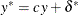#### Adjusting Imputed Classification Variables

For an imputed classification variable, you can specify adjustment parameters for the response level. The SHIFT=option specifies the shift parameter, the SIGMA=option specifies the sigma parameter> 0, and the EVENT=’level’ option identifies the response level.

When the sigma parameter is not specified, the shift parameteris used in all imputations. When you specify a sigma parameter, a simulated shift parameter is generated from the normal distribution that has meanand standard deviationfor each imputationThe next three sections provide details for adjusting imputed binary, ordinal, and nominal response variables.

#### Adjusting Imputed Binary Response Variables

For an imputed binary classification variable Y, the shift parameteris applied to the logit function values for the corresponding response level.

For instance, if Y has binary responses 1 and 2, a simulated logit modelis used to impute the missing response values. For a detailed description of this simulated logit model, see the section Binary Response Logistic Regression.

For an observation that has missing Y and covariates, the predicted probabilities that Y=1 and Y=2 are then given by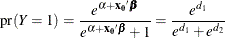where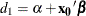and and.

When you provide the shift parametersfor the response Y=1 and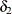for the response Y=2, the predicted probabilities arewhereand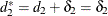.

For example, the following statement specifies the shift parametersand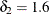:

mnar adjust( y(event='1') / shift=0.8)
adjust( y(event='2') / shift=1.6);


The statement

mnar adjust( y(event='1') / shift=0.8 sigma=0.2);


simulates a shift parameterfrom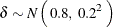in each imputation. Because an adjustment is not specified for Y=2, the corresponding shift parameter is.

#### Adjusting Imputed Ordinal Response Variables

For an imputed ordinal classification variable Y, the shift parameteris applied to the cumulative logit function values for the corresponding response level.

For instance, if Y has ordinal responses 1, 2, …, K, a simulated cumulative logit model that has covariates,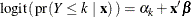is used to impute the missing response values, where k = 1, 2, …, K–1. For a detailed description of this model, see the section Ordinal Response Logistic Regression.

For an observation that has missing Y and covariates, the predicted cumulative probability for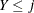, j = 1, 2, …, K–1, is then given bywhereand.

The predicted probabilities for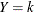areFor an ordinal logistic regression method that has two response levels, the section Adjusting Imputed Binary Response Variables explains how the predicted probabilities are adjusted using shift parameters.

For an ordinal logistic regression method that has more than two response levels, only one classification level can be adjusted. When you provide the shift parameterfor the response level, the predicted probability foris then given bywhere.

The predicted probabilities for the remainingare then adjusted proportionally. When the shift parameteris less than 0, the value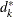can be less than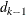for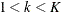. In this case,is set to 0.

#### Adjusting Imputed Nominal Response Variables

For an imputed nominal classification variable Y, the shift parameteris applied to the generalized logit model function values for the corresponding response level.

For instance, if VariableY has nominal responses 1, 2, …, K, a simulated generalized logit model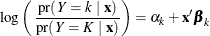is used to impute the missing response values, where k=1, 2, …, K–1. For a detailed description of this model, see the section Nominal Response Logistic Regression.

For an observation with missing Y and covariates, the predicted probability for Y = j, j < K, is then given byandwhereforand.

When you use the shift parameters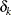for, the predicted probabilities are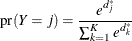where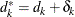.